# if-else Statements & Basic Plotting

## if Statement

• An if statement tells R to do a certain task for a certain case.
• In English, you would say “If <condition> is true, do <steps>!”
• In R, you would say:
if (condition) {
step1
step2
...
}
• condition object should be a logical statement/test or an R expression that evaluates to a single TRUE or FALSE.

• If condition is TRUE,
• R will run all the code that appears between the curly brackets {} following the if statement.
• If condition is FALSE,
• R will skip the code between the curly brackets without running it.

Below is an example of an if statement to make sure a number num is positive.

if (num < 0) {
num <- num * -1
}
• In the case, num < 0 is TRUE:
num <- -2

if (num < 0) {
num <- num * -1
}

num
##  2
• In the case, num < 0 is FALSE:
num <- 4

if (num < 0) {
num <- num * -1
}

num
##  4
• Quiz 1: What will the following code return?
x <- 1
if (3 == 3) {
x <- 2
}
x
• Quiz 2: What will the following code return?
x <- 1
if (TRUE) {
x <- 2
}
x
• Quiz 3: What will the following code return?
x <- 1
if (x == 1) {
x <- 2
if (x == 1) {
x <- 3
}
}
x

## else Statement

• if statement tells R what to do when the condition is TRUE.
• else statement tells R what to do when the condition is FALSE.
if (condition) {
Plan A
} else {
Plan B
}
• Example: Write a function for rounding a number to the nearest whole number.
a <- 3.14
• Isolate the decimal component with trunc() function.
decimal <- a - trunc(a)
decimal
##  0.14
• Use if else to round the number (up or down):
if (decimal >= 0.5) {
a <- trunc(a) + 1
} else {
a <- trunc(a)
}

a
##  3
• Write the rounding function:
my_round <- function(a) {
decimal <- a - trunc(a)
if (decimal >= 0.5) {
a <- trunc(a) + 1
} else {
a <- trunc(a)
}
return(a)
}
my_round(3.14)
##  3
round(3.14)
##  3
my_round(4.9)
##  5
round(4.9)
##  5
• Another example
a <- 1
b <- 1

if (a > b) {
print("A wins!")
} else if (a < b) {
print("B wins!")
} else {
print("Tie.")
}
##  "Tie."

## Basic Plots

### Histogram

cars
hist(x = cars$speed)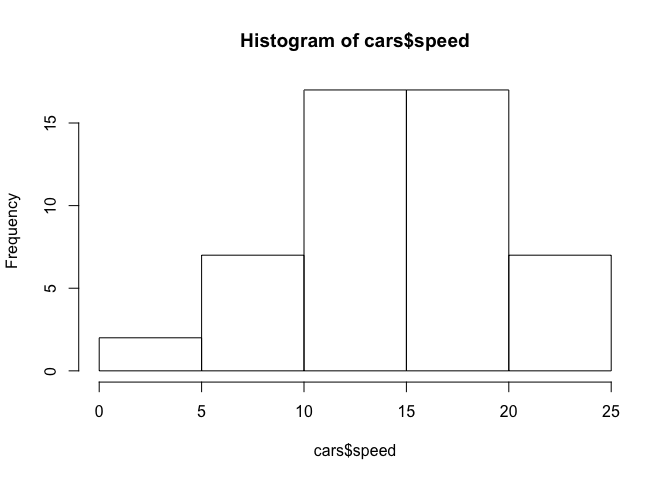• Change the plot title hist(x = cars$speed, main = "Histogram of Speed")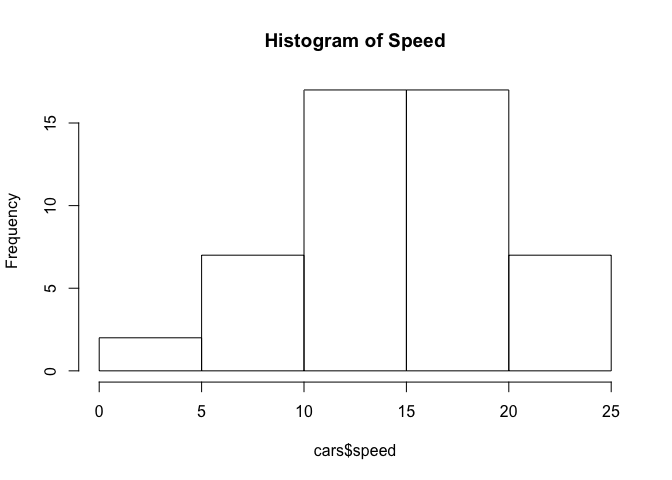• Change x-axis label
hist(x = cars$speed, main = "Histogram of Speed", xlab = "Speed (mph)")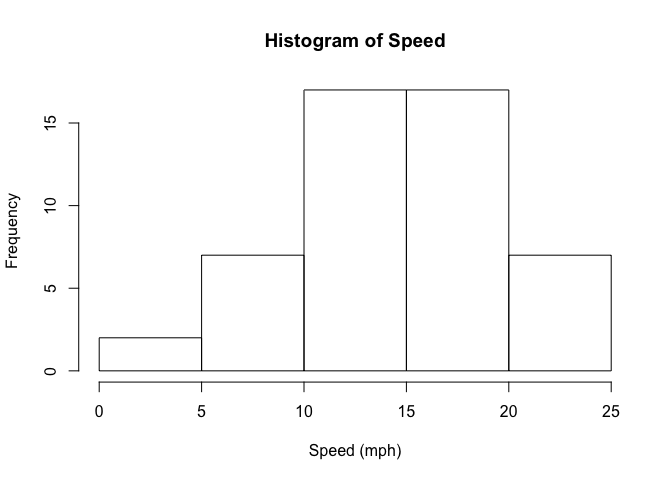• Change the y-axis (from frequency) to probability/density hist(x = cars$speed, main = "Histogram of Speed", xlab = "Speed (mph)",
probability = TRUE)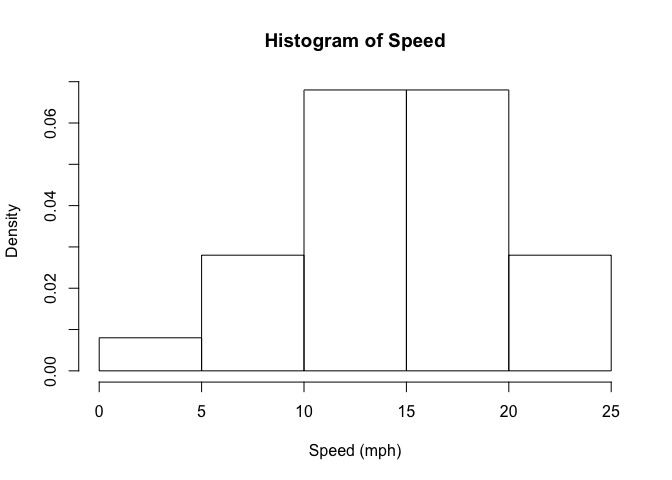• Change how the histogram is graphed (by changing the column width):
• The argument breaks in hist() can take one of the following:
• a vector giving the breakpoints between histogram cells,
• a function to compute the vector of breakpoints,
• a single number giving the number of cells for the histogram,
• a character string naming an algorithm to compute the number of cells (see ‘Details’),
• a function to compute the number of cells.
# a vector giving the breakpoints between histogram cells
hist(x = cars$speed, main = "Histogram of Speed", xlab = "Speed (mph)", probability = TRUE, breaks = c(0, 4, 8, 12, 16, 20, 24, 28, 32))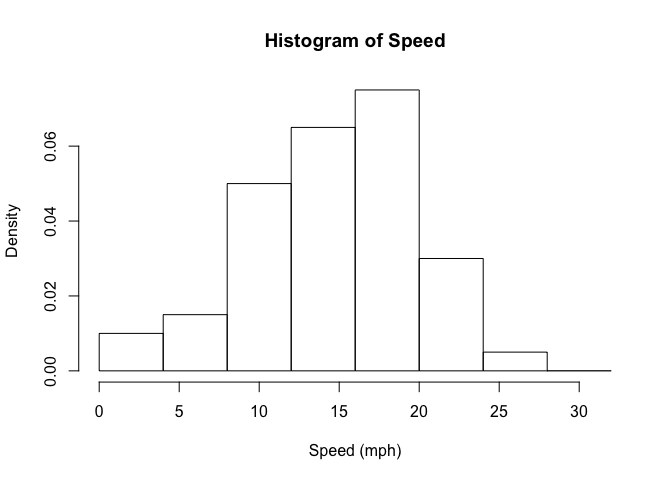# a function to compute the vector of breakpoints hist(x = cars$speed, main = "Histogram of Speed", xlab = "Speed (mph)",
probability = TRUE, breaks = seq(from = 0, to = 30, by = 3))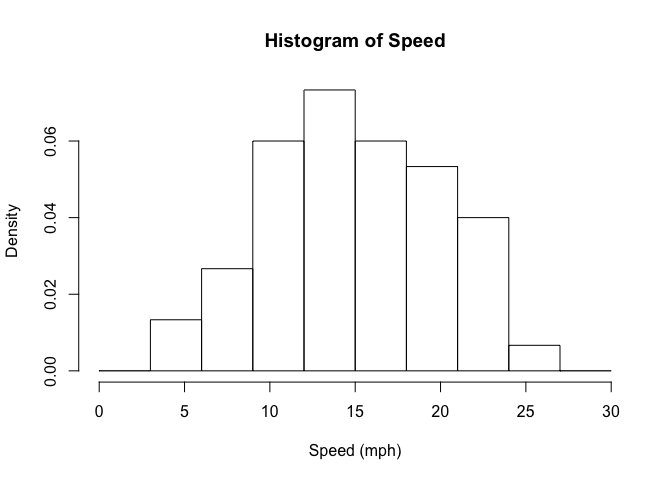# a single number giving the number of cells for the histogram
hist(x = cars$speed, main = "Histogram of Speed", xlab = "Speed (mph)", probability = TRUE, breaks = 10)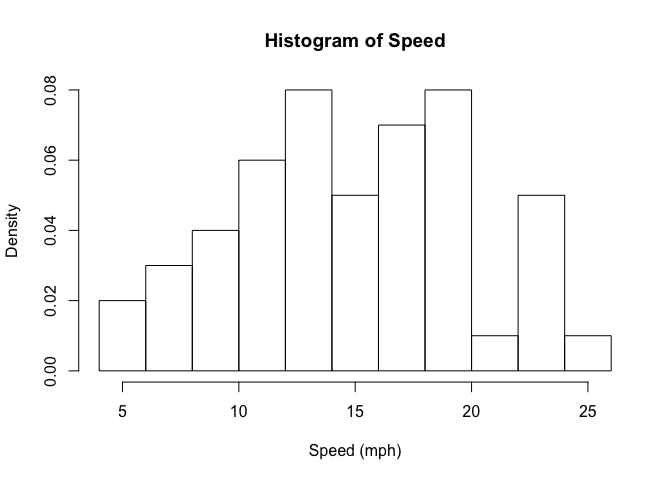# a single number giving the number of cells for the histogram hist(x = cars$speed, main = "Histogram of Speed", xlab = "Speed (mph)",
probability = TRUE, breaks = "Freedman-Diaconis")# a function to compute the number of cells
hist(x = cars$speed, main = "Histogram of Speed", xlab = "Speed (mph)", probability = TRUE, breaks = 5*2)• Let’s add some color to this histogram! hist(x = cars$speed, main = "Histogram of Speed", xlab = "Speed (mph)",
probability = TRUE, breaks = seq(from = 0, to = 30, by = 3),
col = "darkorange")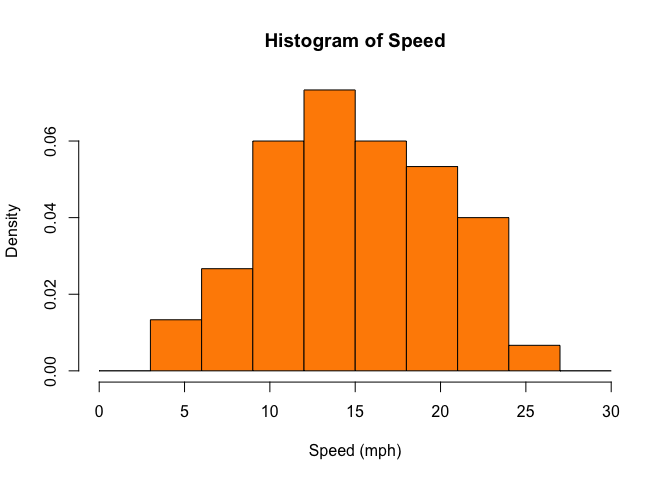• A bit too much?
hist(x = cars$speed, main = "Histogram of Speed", xlab = "Speed (mph)", probability = TRUE, breaks = seq(from = 0, to = 30, by = 3), border = "dodgerblue")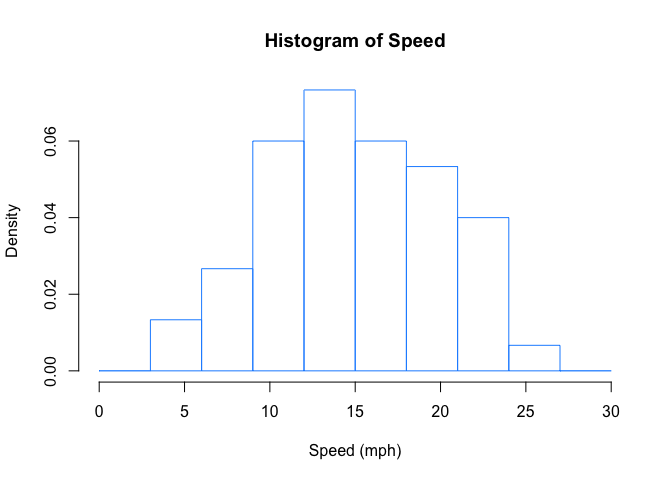• Make it looks “professional”! hist(x = cars$speed, main = "Histogram of Speed", xlab = "Speed (mph)",
probability = TRUE, breaks = seq(from = 0, to = 30, by = 3),
col = "lightgrey")
box()
grid()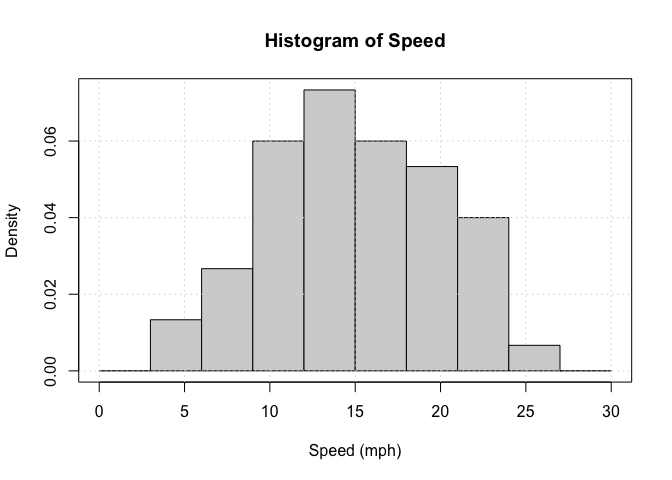### Boxplot

boxplot(x = cars$speed)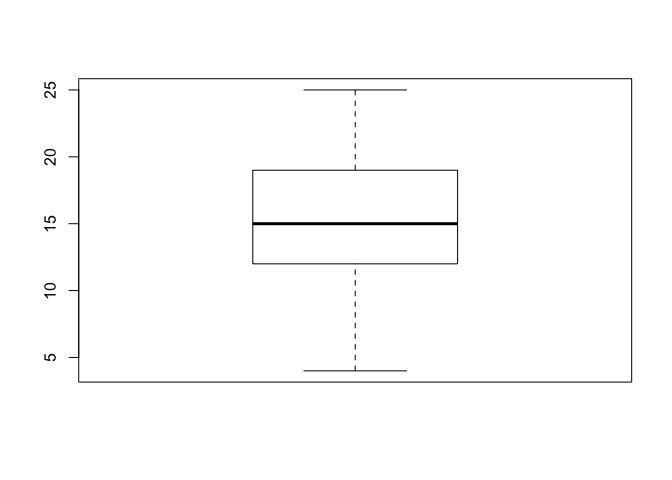• Add title and axis label boxplot(x = cars$speed, main = "Boxplot of Speed", ylab = "Speed (mph)")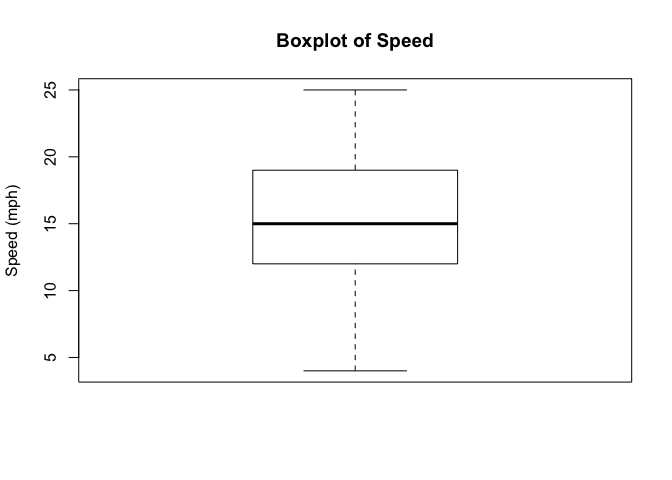• Change the orientation of the boxplot
boxplot(x = cars$speed, main = "Boxplot of Speed", xlab = "Speed (mph)", horizontal = TRUE)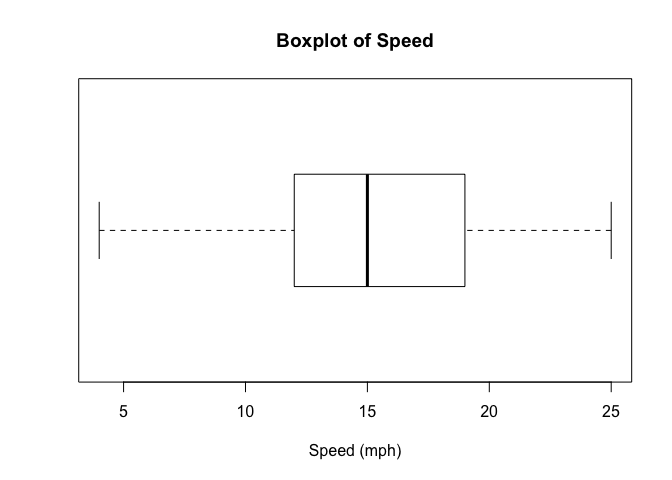### Scatterplot plot(x = cars$speed, y = cars$dist)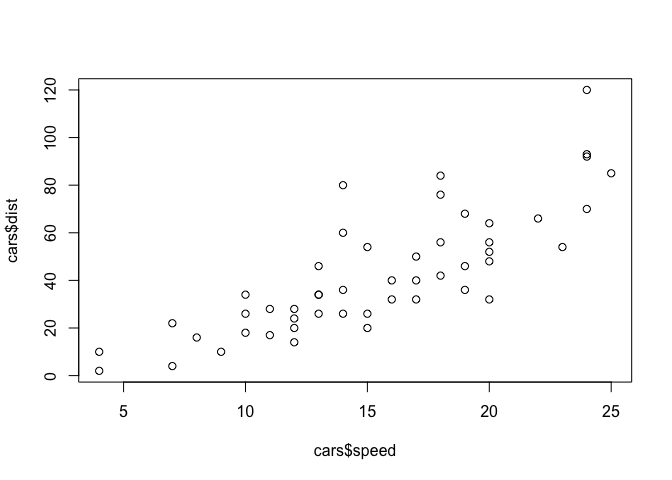• Adding plot title, axis labels plot(x = cars$speed, y = cars$dist, main = "Car Speed vs. Stopping Distance", xlab = "Speed (mph)", ylab = "Stopping Distance (ft)")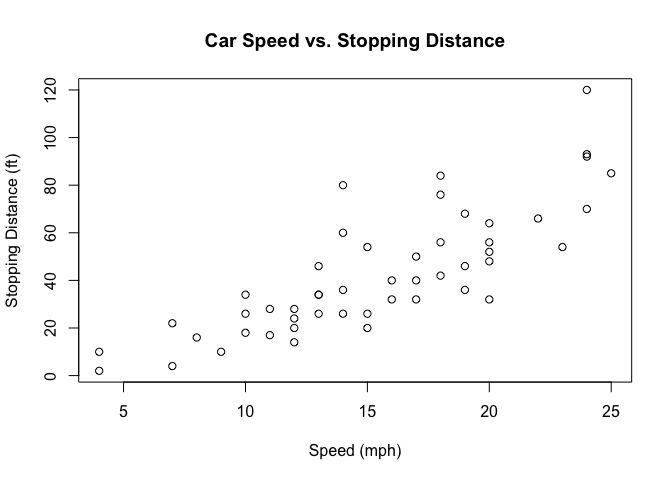• Add color, change symbol, and other modifications. • See more details here. plot(x = cars$speed, y = cars\$dist, main = "Car Speed vs. Stopping Distance",
xlab = "Speed (mph)", ylab = "Stopping Distance (ft)",
col = "dodgerblue", pch = 19)
grid()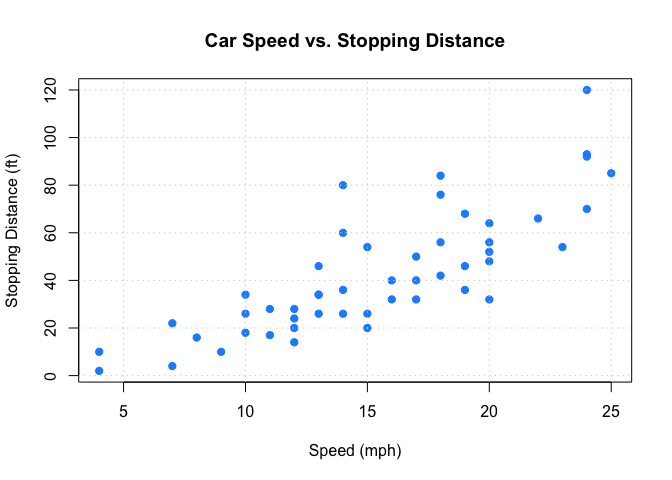• Do you notice a difference in the code segment above and below?
plot(dist ~ speed, data = cars,
main = "Car Speed vs. Stopping Distance",
xlab = "Speed (mph)", ylab = "Stopping Distance (ft)",
col = "dodgerblue", pch = 19)
grid()